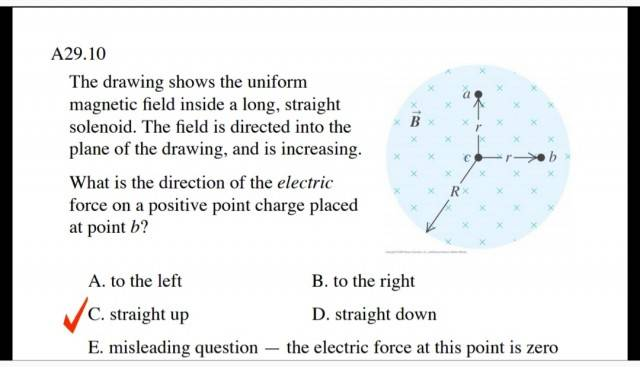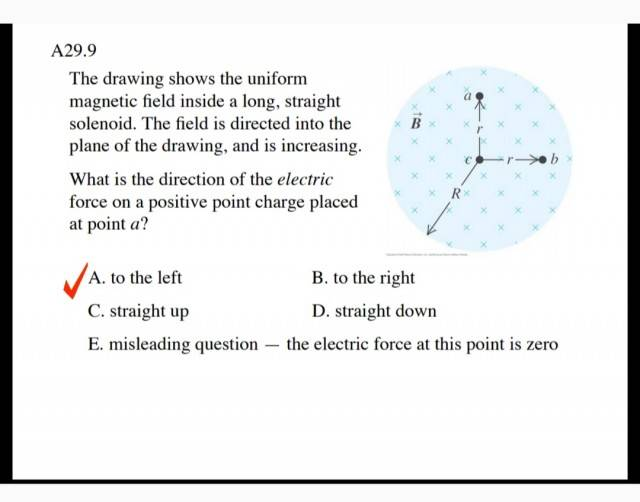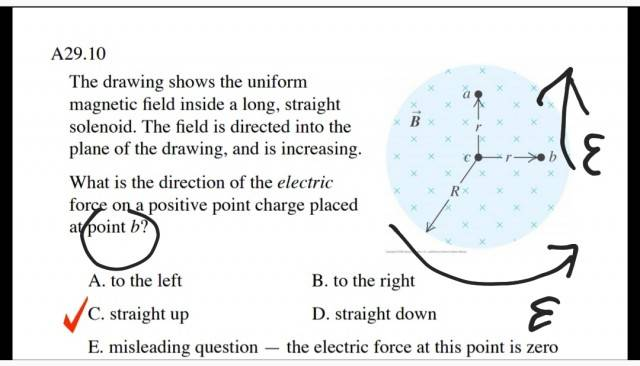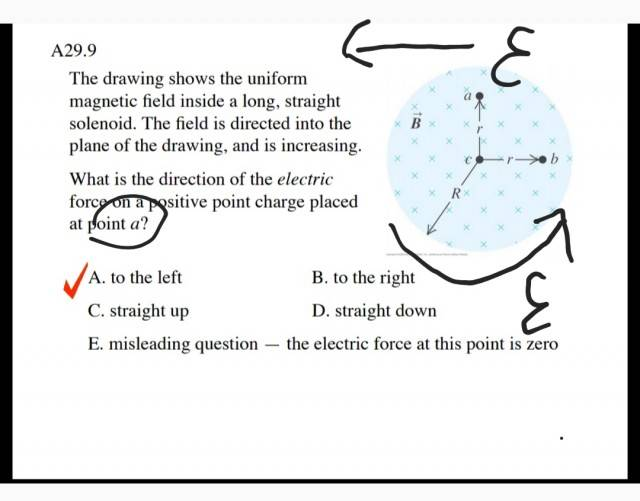# Electric Force on a Charge in a Solenoid

## Homework StatementSince i only know the field direction, increasing go into page. Why the answer is C?The R and r on the pic is respected to what?

## The Attempt at a Solution

Right hand rule : finger tip = current
Thumbs = force
Palm = B

Or
Finger tip = velocity
Thumbs = current
Palm = B

#### Attachments

Homework Helper
Gold Member
The key word here in the statement of the problem is "increasing" and they should say "increasing with time". The question involves the Faraday EMF that occurs. The changing magnetic flux creates an EMF. They basically are asking the direction of the induced ## E ## of the EMF.

•Helly123
Could it be like this?Force direction the same as Emf's ?

#### Attachments

Homework Helper
Gold Member
That is correct. The EMF is the integral of an induced electric field along the path. The electric field ## \vec{E}=\vec{E}_{induced} ## is what the positive charge responds to, and experiences a force ## \vec{F}=q(\vec{E}+\vec{v} \times \vec{B}) ##. ## \\ ## (And of course, when they say "placed at point b", etc. that means ## \vec{v}=0 ## so that ## \vec{v} \times \vec{B}=0 ## ).

•Helly123
That is correct. The EMF is the integral of an induced electric field along the path. The electric field ## \vec{E}=\vec{E}_{induced} ## is what the positive charge responds to, and experiences a force ## \vec{F}=q(\vec{E}+\vec{v} \times \vec{B}) ##. ## \\ ## (And of course, when they say "placed at point b", etc. that means ## \vec{v}=0 ## so that ## \vec{v} \times \vec{B}=0 ## ).
•